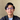# How to Generate Unique ID in JavaScript

December 22, 2019

There are several ways to generate unique identifier in JavaScript. This could very useful in many cases, such as rendering list efficiently, or storing documents or records in database.

## Using UUID

UUID is the abbreviation of univerally unique identifier, which is an identification number to uniquely identify something. The main idea of this thing is everytime we generate this numbers, it will be universally unique, which means no one could generate the exact same id as yours.

I personally prefer this approach in any case. In JavaScript, we can use a library called `uuid` to generate UUID.

``\$ npm install uuid``
``````const uuidv4 = require("uuid/v4")

uuidv4()``````

UUID has several versions, but the version that appropriate for generating unique id is version 4. And, that code will generate something like this.

``1b9d6bcd-bbfd-4b2d-9b5d-ab8dfbbd4bed``

## Using Math.random

Math.random is a JavaScript built-in function which allows us to generate a random number. Which means that everytime we run it, it will return a unique combination of numbers.

``Math.floor(Math.random() * 100)``

`Math.random` always returns a decimal number, so we need to rounding off that number first. By multiply it with 100, it will return any number between 0 to 99. You can increase the number if you want to get better result.

Result:

``52``

## Using Date.now

Date.now is another JavaScript built-in function which allows us to get the number of miliseconds elapsed since January 1, 1970.

``Date.now()``

Result:

``1576996323453``